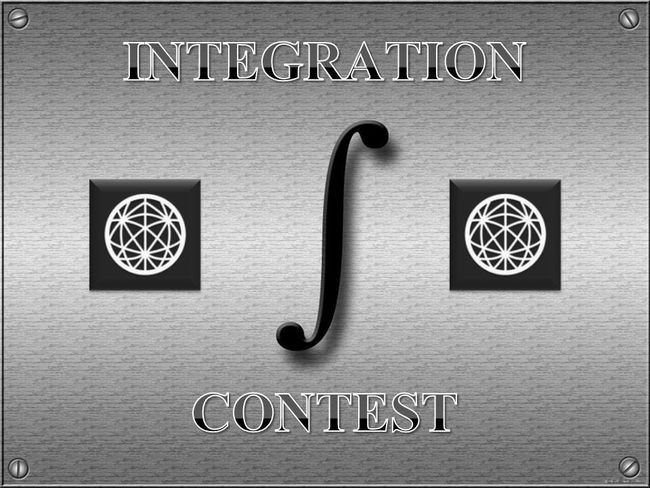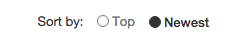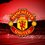# Brilliant Integration Contest - Season 3Hi Brilliant! Here is the most awaited Integration contest! See Season 1 and Season 2.

The aims of the Integration contest are to improve skills in the computation of integrals, to learn from each other as much as possible, and of course to have fun. Anyone here may participate in this contest.

The rules are as follows:

• I will start by posting the first problem. If there is a user solves it, then they must post a new one.

• You may only post a solution of the problem below the thread of problem and post your proposed problem in a new thread. Put them separately.

• Only make substantial comment that will contribute to the discussion.

• Make sure you know how to solve your own problem before posting it in case there is no one can answer it within 48 hours, then you must post the solution and you have a right to post another problem.

• If the one who solves the last problem does not post his/her own problem after solving it within a day, then the one who has a right to post a problem is the last solver before him/her.

• The scope of questions is only computation of integrals either definite or indefinite integrals.

• You are NOT allowed to post a multiple integrals problem.

• It is NOT compulsory to post original problems. But make sure it has not been posted on brilliant.

• Do not copy questions from last year's contest. If anyone found to do so he/she will be banned from taking further part in this contest

• Direct problems on contour integration are not allowed. However, solutions which use contour integration are allowed.

• Also don't forget to upvote good problems and solutions!

Format your post is as follows:

 1 2 3 4 5 6 7 **SOLUTION OF PROBLEM xxx (number of problem) :** **[Post your solution here]** **PROBLEM xxx (number of problem) :** **[Post your problem here]** 

The comments will be easiest to follow if you sort by "Newest":4 years, 7 months ago

This discussion board is a place to discuss our Daily Challenges and the math and science related to those challenges. Explanations are more than just a solution — they should explain the steps and thinking strategies that you used to obtain the solution. Comments should further the discussion of math and science.

When posting on Brilliant:

• Use the emojis to react to an explanation, whether you're congratulating a job well done , or just really confused .
• Ask specific questions about the challenge or the steps in somebody's explanation. Well-posed questions can add a lot to the discussion, but posting "I don't understand!" doesn't help anyone.
• Try to contribute something new to the discussion, whether it is an extension, generalization or other idea related to the challenge.

MarkdownAppears as
*italics* or _italics_ italics
**bold** or __bold__ bold
- bulleted- list
• bulleted
• list
1. numbered2. list
1. numbered
2. list
Note: you must add a full line of space before and after lists for them to show up correctly
paragraph 1paragraph 2

paragraph 1

paragraph 2

[example link](https://brilliant.org)example link
> This is a quote
This is a quote
    # I indented these lines
# 4 spaces, and now they show
# up as a code block.

print "hello world"
# I indented these lines
# 4 spaces, and now they show
# up as a code block.

print "hello world"
MathAppears as
Remember to wrap math in $$ ... $$ or $ ... $ to ensure proper formatting.
2 \times 3 $2 \times 3$
2^{34} $2^{34}$
a_{i-1} $a_{i-1}$
\frac{2}{3} $\frac{2}{3}$
\sqrt{2} $\sqrt{2}$
\sum_{i=1}^3 $\sum_{i=1}^3$
\sin \theta $\sin \theta$
\boxed{123} $\boxed{123}$

Sort by:

Problem 6:

Prove that $\int_0^\infty \frac{\ln x}{(x+a)^2 + b^2}\,dx \; = \; \tfrac{1}{b}\,\tan^{-1}\tfrac{b}{a}\,\ln\sqrt{a^2+b^2}$ for all $a,b > 0$.

This problem has been solved by Aditya Sharma.

- 4 years, 7 months ago

Solution to problem 6 :

Let us denote the integral by $I$ .

$\displaystyle I = \int_0^\infty \frac{\ln x}{(x+a)^2+b^2}dx = \int_0^\infty \frac{\ln x}{(x+a+ib)(x+a-ib)}$

With the substitution $xy=a^2+b^2$ we have,

$\displaystyle I=\ln(a^2+b^2)\int_0^\infty \frac{dy}{y^2+2ay+a^2+b^2} - \underbrace{\int_0^\infty \frac{\ln y}{(y+a)^2+b^2}dy}_I$

$\displaystyle I=\ln(\sqrt{a^2+b^2})\int_0^\infty \frac{dx}{(x+a)^2+b^2} = \frac{1}{b}\tan^{-1}\frac{b}{a}\ln\sqrt{a^2+b^2}$.

- 4 years, 7 months ago

As an alternative to Aditya's proof...

Consider the cut plane $\mathbb{C} \backslash [0,\infty)$, so that $0 < \mathrm{Arg}\,z < 2\pi$ for all $z$, and consider the integral of $f(z) \; = \; \frac{(\log z)^2}{(z+a)^2 + b^2}$ around the keyhole contour $\gamma_1 + \gamma_2 - \gamma_3 - \gamma_4$, where

• $\gamma_1$ is the line segment $z \,=\, x e^{0i}$, for $\varepsilon < x < R$, just above the cut,
• $\gamma_2$ is the circular contour $z \,=\, Re^{i\theta}$ for $0 < \theta < 2\pi$,
• $\gamma_3$ the the line segment $z \,=\, x e^{2\pi i}$, for $\varepsilon < x < R$, just below the cut,
• $\gamma_4$ is the circular contour $z \,=\, \varepsilon e^{i\theta}$ for $0 < \theta < 2\pi$.

where we assume that $0 < \varepsilon < \sqrt{a^2+b^2} < R$. Now \begin{aligned} \int_{\gamma_1} f(z)\,dz & = \int_\varepsilon^R \frac{(\ln x)^2}{(x+a)^2 + b^2}\,dx \\ \int_{\gamma_3} f(z)\,dz & = \int_\varepsilon^R \frac{(\ln x + 2\pi i)^2}{(x+a)^2 + b^2}\,dx \\ \left(\int_{\gamma_1} - \int_{\gamma_3}\right)\,f(z)\,dz & = \int_{\varepsilon}^R \frac{4\pi^2 - 4\pi i \ln x}{(x+a)^2 + b^2}\,dx \end{aligned} We also note that $\int_{\gamma_2} f(z)\,dz \; = \; O\big(\tfrac{\ln^2R}{R}\big) \hspace{2cm} \int_{\gamma_4} f(z)\,dz \; = \; O\big(\varepsilon \ln^2\varepsilon\big)$ as $R \to \infty$ and $\varepsilon \to 0$. Letting $R \to \infty$ and $\varepsilon \to 0$, we deduce that $\int_0^\infty \frac{4\pi^2 - 4\pi i \ln x}{(x+a)^2 + b^2}\,dx \; = \; 2\pi i\Big[ \mathrm{Res}_{z=-a+ib} f(z) \,+\, \mathrm{Res}_{z=-a-ib} f(z)\Big]$ Now $f(z)$ has simple poles at each of $-a \pm ib$, and \begin{aligned} \mathrm{Res}_{z = -a\pm ib} f(z) & = \frac{\Big(\ln\sqrt{a^2+b^2} + i\pi \mp i\tan^{-1}\frac{b}{a}\Big)^2}{\pm 2ib} \\ \mathrm{Res}_{z = -a+ib} f(z) + \mathrm{Res}_{z = -a-ib} f(z) & = \frac{-4i(\ln\sqrt{a^2+b^2}+i\pi)\tan^{-1}\frac{b}{a}}{2ib} \\ & = - \frac{2}{b}\tan^{-1}\frac{b}{a}(\ln\sqrt{a^2+b^2} + i\pi) \end{aligned} and hence $\int_0^\infty \frac{4\pi^2 - 4\pi i \ln x}{(x+a)^2 + b^2}\,dx \; = \; \frac{4\pi}{b}\tan^{-1}\frac{b}{a}\big(\pi - i\ln\sqrt{a^2+b^2}\big)$ Taking imaginary parts of this equation gives the result.

- 4 years, 7 months ago

Problem 16:

Evaluate the integral $\int_0^1 \frac{x^{a-1}(1-x)^{b -1}}{(x+p)^{a+b}}\,dx$ for all $a,b,p > 0$.

This problem has been solved by Aditya Sharma.

- 4 years, 7 months ago

If we use the substitution, $\displaystyle x=\frac{1}{u+1}$ then

$\displaystyle I =\int_0^\infty \frac{u^{b-1}}{(up+p+1)^{a+b}}\,du$

Again using the substitution, $\displaystyle u=y\left(\frac{p+1}{p}\right)$ the integral transforms to,

$\displaystyle I =\frac{1}{p^b(1+p)^a}\int_0^\infty \frac{y^{b-1}}{(1+y)^{a+b}}\,dy = \left(\frac{\beta(a,b)}{p^b(1+p)^a}\right)$

- 4 years, 7 months ago

The substitution $z = \frac{(p+1)x}{p+x}$ Is even more direct. Not a hard problem, but a very elegant result.

- 4 years, 7 months ago

Yes, Although this substitution emerges by combining the chain of substitutions used, or it's tough to put it directly without knowing why it is the reqd. substitution.

- 4 years, 7 months ago

Problem 14 : Prove That

$\int_{0}^{\infty} \ln x\left[\ln \left( \dfrac{x+1}{2} \right) - \dfrac{1}{x+1} - \psi \left( \dfrac{x+1}{2} \right) \right] \mathrm{d}x = \dfrac{\ln^2 2}{2}+\ln2\cdot\ln\pi-1$

Notation : $\psi(x)$ denotes the Digamma Function.

This problem has been solved by Mark Hennings.

- 4 years, 7 months ago

This is a really nice use of previous results of this round of the contest!

Starting with the identity $\psi(x) \; = \; \ln x - \frac{1}{2x} - 2\int_0^\infty \frac{t}{(t^2+x^2)(e^{2\pi t}-1)}\,dt$ we deduce that \begin{aligned} \ln\big(\tfrac{x+1}{2}\big) + \tfrac{1}{x+1} - \psi\big(\tfrac{x+1}{2}\big) & = 2\int_0^\infty \frac{t}{\big(t^2 + \frac14(x+1)^2\big)(e^{2\pi t} - 1)}\,dt \\ & = 8\int_0^\infty \frac{t}{(4t^2 + (x+1)^2)(e^{2\pi t} - 1)}\,dt \\ & = 2\int_0^\infty \frac{t}{(t^2 + (x+1)^2)(e^{\pi t}-1)}\,dt \end{aligned} and hence \begin{aligned} \int_0^\infty \ln x & \left(\ln\big(\tfrac{x+1}{2}\big) + \tfrac{1}{x+1} - \psi\big(\tfrac{x+1}{2}\big)\right)\,dx \\ & = 2\int_0^\infty \left(\int_0^\infty \frac{\ln x}{(x+1)^2 + t^2}\,dx\right)\,\frac{t}{e^{\pi t}-1}\,dt \\ & = 2\int_0^\infty \left(\frac{1}{t} \tan^{-1}t \ln\sqrt{t^2 + 1}\right)\,\frac{t}{e^{\pi t}-1}\,dt \; = \; \int_0^\infty \frac{\tan^{-1}t \ln(t^2+1)}{e^{\pi t} - 1}\,dt \\ & = \tfrac12\ln^22 + \ln 2 \ln\pi - 1 \end{aligned} using the results of Problems $5$ and $6$.

- 4 years, 7 months ago

Yes indeed! This integral arose from my attempt to find an alternate solution of Problem 5 :) The first identity can be proved (using real analysis) by differentiating the identity $\int_{0}^{\infty} \dfrac{\log(1-e^{-2a\pi x})}{1+x^2} \mathrm{d}x = \pi \left[\dfrac{1}{2} \log (2a\pi ) + a(\log a - 1) - \log(\Gamma(a+1)) \right]$ wrt $a$. I have proved it here.

- 4 years, 7 months ago

I have posted a question on Math Stack Exchange asking for alternate methods to evaluate the above integral (independently of Problems $5$ and $6$) here. If we can evaluate it using other methods, it'll also give us an alternate solution for Problem $5$.

- 4 years, 7 months ago

Problem 1:

Show that: $\int_{0}^{1}\frac{\cosh(a\log(x))\log(1+x)}{x}dx=\frac{1}{2a}(\pi \csc(\pi a)-\frac{1}{a}), \;\ a<1$

This problem has been solved by Aditya Sharma.

- 4 years, 7 months ago

If we denote the integral by $I$ & using $2cosh(a\ln x)=x^a+x^{-a}$ & $\displaystyle \ln(1+x)=\sum_{n\ge 1}\frac{(-1)^{n-1}}{n}x^n$ the integral can be transformed into,

$\displaystyle I = \sum_{n\ge 1} \frac{(-1)^{n-1}}{n} \int_0^1 (x^{n+a-1}+x^{n-a-1})=\sum_{n\ge 1} \frac{(-1)^{n-1}}{n}(\frac{1}{n-a}+\frac{1}{n+a})=\frac{1}{2a}\sum_{n\ge 1} (-1)^{n-1} (\frac{1}{n-a}-\frac{1}{n+a})$

For removing the alternating factor $(-1)^{n-1}$ this can be written as ,

$\displaystyle 2a I = \sum_{n\ge 1} (\frac{1}{n-a}-\frac{1}{n+a})-2\sum_{n\ge 1} (\frac{1}{2n-a}-\frac{1}{2n+a})$

which simplifies to ,

$\displaystyle 2a I = \sum_{n\ge 1} (\frac{1}{n-a}-\frac{1}{n+a}-\frac{1}{n-\frac{a}{2}}+\frac{1}{n+\frac{a}{2}})$

Using $\displaystyle H_a = \sum_{n\ge 1}(\frac{1}{n}-\frac{1}{n+a})$ we have,

$\displaystyle I=\frac{1}{2a}(H_a-H_{-a}-H_{\frac{a}{2}}+H_{-\frac{a}{2}})$

Again using reflection formula for harmonic numbers as $a<1$

i.e. $\displaystyle H_{1-a}-H_a = \pi \cot(\pi a)-\frac{1}{a}+\frac{1}{1-a}$ it simplifies to,

$\displaystyle I = \frac{1}{2a} (-\pi\cot(\pi a)+\pi\cot(\pi\frac{a}{2})-\frac{1}{a}) = \frac{1}{2a}(\pi\csc(\pi a)-\frac{1}{a})$

- 4 years, 7 months ago

Problem 5:

Prove that:

${\large\int}_0^\infty\frac{\ln\left(x^2+1\right)\,\arctan x}{e^{\pi x}-1}dx=\frac{\ln^22}2+\ln2\cdot\ln\pi-1.$

This problem has been solved by Mark Hennings.

- 4 years, 7 months ago

Solution of Problem 5

Starting with the Hermite formula for the generalized Riemann zeta function: $\zeta(s,a) \; = \; \tfrac12a^{-s} + a^{1-s}(s-1)^{-1} + 2\int_0^\infty \frac{(a^2+y^2)^{-\frac12s} \, \sin\big(s \tan^{-1}\frac{y}{a}\big)}{e^{2\pi y} - 1}\,dy$ we have \begin{aligned} \zeta(s,\tfrac12) & = 2^{s-1}\left(1 + \tfrac{1}{s-1}\right) + 2\int_0^\infty \frac{(\frac14 + y^2)^{-\frac12s}\,\sin\big(s \tan^{-1}2y\big)}{e^{2\pi y}-1}\,dy \\ & = 2^{s-1}\frac{s}{s-1} + 2^{s+1}\int_0^\infty \frac{(1 + 4y^2)^{-\frac12s} \, \sin\big(\tan^{-1}2y\big)}{e^{2\pi y} - 1}\,dy \\ & = 2^{s-1}\frac{s}{s-1} + 2^s \int_0^\infty \frac{(1+t^2)^{-\frac12s}\,\sin\big(s \tan^{-1}t\big)}{e^{\pi t}-1}\,dt \end{aligned} so that $F(s) \; = \; \int_0^\infty \frac{(1+t^2)^{-\frac12s}\,\sin(s \tan^{-1}t\big)}{e^{\pi t}-1}\,dt \; = \; 2^{-s}\zeta(s,\tfrac12) - \frac{s}{2(s-1)}$ Now since \begin{aligned} F''(s) & = \frac{\partial^2}{\partial s^2}\int_0^\infty \frac{(1 + t^2)^{-\frac12s} \, \sin(s \tan^{-1}t\big)}{e^{\pi t}-1}\,dt \\ & = \int_0^\infty \frac{(1+t^2)^{-\frac12s}}{e^{\pi t} - 1}\left\{ \begin{array}{l} \tfrac14\big(\ln(1+t^2)\big)^2 \sin(s\tan^{-1}t) - \ln(1 + t^2) \tan^{-1}t \cos\big(s \tan^{-1}t\big) \\ - \big(\tan^{-1}t\big)^2 \sin\big(s \tan^{-1}t\big)\end{array}\right\}\,dt \end{aligned} we deduce that $F''(0) \; = \; -\int_0^\infty \frac{\ln(1+t^2) \tan^{-1}t}{e^{\pi t}-1}\,dt$ and hence that the desired integral is $\int_0^\infty \frac{\ln(1+t^2) \tan^{-1}t}{e^{\pi t}-1}\,dt \; = \; -F''(0) \; = \; \frac{\partial^2}{\partial s^2}\Big[\tfrac{s}{2(s-1)} - 2^{-s}\zeta(s,\tfrac12)\Big] \Big|_{s=0}$ which simplifies to give $\int_0^\infty \frac{\ln(1+t^2) \tan^{-1}t}{e^{\pi t}-1}\,dt \; = \; -1 - \tfrac12\ln^22 + \ln2 \cdot \ln2\pi \; = \; -1 + \tfrac12\ln^22 + \ln2\ln\pi$

- 4 years, 7 months ago

Problem 8:

Show that $\int_0^{\frac12\pi} \ln^2\big(a\sin\theta\big)\,d\theta \; = \; \tfrac{1}{24}\pi^3 + \tfrac{1}{6}\pi\Big[\ln^2\big(\tfrac{2}{a}\big) - 2\ln\big(\tfrac{2}{a}\big)\ln\big(\tfrac{a}{2}\big)\Big]$ for any $a > 0$.

This problem has been solved by Jasper Braun.

- 4 years, 7 months ago

Solution to Problem 8: $\displaystyle I=\int_0^\frac{\pi}{2}\ln^2{a}+2\ln{a}\ln{\sin{x}}+\ln^2{\sin{x}}dx=\frac{\pi\ln^2{a}}{2}-\ln{a}\pi\ln{2}+\int_0^\frac{\pi}{2}\ln^2{\sin{x}}dx$

$\displaystyle\int_0^\frac{\pi}{2}\ln^2{\sin{x}}dx = \int_0^1\frac{\ln^2{x}}{\sqrt{1-x^2}}dx\quad\text{(by }u= \sin{x})$

$\displaystyle\text{Now using }B(m,n) = 2\int_0^1x^{2m-1}(1-x^2)^{n-1}dx \text{ and thus }\int_0^1\frac{\ln^2{x}}{\sqrt{1-x^2}}dx = \frac{d^2}{dm^2}\frac{B(m,\frac{1}{2})}{2*4}\big|_\frac{1}{2}$

$\displaystyle\text{Using }\Gamma'(m) = \Gamma(m)\psi(m),\qquad\Gamma''(m) = \Gamma(m)\psi(m)^2+\Gamma(m)\psi^{(1)}(m)$

$\displaystyle\frac{\sqrt{\pi}}{8}\frac{d^2}{dm^2}\frac{\Gamma(m)}{\Gamma(m+\frac{1}{2})}\Big|_\frac{1}{2} = \frac{\pi\ln^2{2}}{2}+\frac{\pi\zeta(2)}{4}$

$\displaystyle I=\boxed{\frac{\pi^3}{24}+\frac{\pi\ln^2{2}}{2}+\frac{\pi\ln^2{a}}{2}-\pi\ln{a}\ln{2}}$

- 4 years, 7 months ago

Problem 13:

Evaluate $\int_0^{2\pi} e^{\cos\theta} \cos\big(n\theta - \sin\theta\big)\,d\theta$ for any integer $n$.

This problem has been solved by Ishan Singh.

- 4 years, 7 months ago

It's simplest using contour integration...

Note that $\int_0^{2\pi} e^{\cos\theta}\,\cos(\sin\theta - n\theta)\,d\theta \; = \; \mathfrak{Re}\left(\int_0^{2\pi} e^{\cos\theta} e^{i(\sin\theta - n\theta)}\,d\theta\right) \; = \; \mathfrak{Re}\left(\int_0^{2\pi} e^{e^{i\theta} - in\theta}\,d\theta\right)$ With the substitution $z = e^{i\theta}$, this becomes the contour integral $\mathfrak{Re}\left(\int_{|z|=1}e^z z^{-n} \frac{dz}{iz}\right) \; = \; \mathfrak{Re}\left(\frac{1}{i}\int_{|z|=1}\frac{e^z}{z^{n+1}}\,dz\right) \; = \; 2\pi\mathfrak{Re}\left(\mathrm{Res}_{z=0} \frac{e^z}{z^{n+1}}\right) \; = \; \left\{ \begin{array}{ll} \frac{2\pi}{n!} & n \ge 0 \\ 0 & n < 0 \end{array} \right.$

- 4 years, 7 months ago

$\text{I} = \int_{0}^{2 \pi} e^{\cos t} \cos (nt - \sin t) \ \mathrm{d}t = \Re{ \left(\int_{0}^{2 \pi} e^{i n t} \cdot e^{(\cos t - i \sin t)} \ \mathrm{d}t \right)}$

$= \Re{ \left(\int_{0}^{2 \pi} e^{i n t} \cdot e^{\Large e^{ -i t}} \ \mathrm{d}t \right)}$

$= \dfrac{2 \pi}{n!} + \Re{ \left(\sum_{r \geq 0 \ ; \ r \neq n} \left( \dfrac{1}{r!} \int_{0}^{2 \pi} e^{i t (n-r)} \ \mathrm{d}t \right) \right)}$

Since last integral is $0$, we have,

$\text{I} = \dfrac{2 \pi}{n!}$

If $n$ is negative, we can write $n = -k \ ; \ k \in \mathbb{Z}^+$, so that,

$\text{J} = \int_{0}^{2 \pi} e^{\cos t} \cos (kt + \sin t) \ \mathrm{d}t$

By the above method, we can similarly show that $\text{J} = 0$.

Therefore,

$\int_{0}^{2 \pi} e^{\cos t} \cos (nt - \sin t) \ \mathrm{d}t = \left\{ \begin{array}{ll} \frac{2\pi}{n!} & n \ge 0 \\ 0 & n < 0 \end{array} \right.$

- 4 years, 7 months ago

Problem 2:

Evaluate $\large \displaystyle \int_{0}^{1} \frac{(x^{2}+1)\tan^{-1}3x}{x} \, dx$.

This problem has been solved by Aditya Sharma.

- 4 years, 7 months ago

The integral can be written as : $\displaystyle I=\underbrace{\int_0^1 x\tan^{-1} (3x) dx}_{I_1}+\underbrace{\int_0^3 \frac{\tan^{-1}x}{x}dx}_{I_2}$

A simple integration by parts would yield $\displaystyle I_1 = \frac{5}{9}\tan^{-1}3 - \frac{1}{6}$

$I_2$ is the Inverse Tangent Integral and we represent it by dilogarithms.

$\displaystyle Ti_2(3)=\frac{1}{2i}(Li_2(3i)-Li_2(-3i))$

So, $\displaystyle I = \frac{5}{9}\tan^{-1}3 - \frac{1}{6} +\frac{1}{2i}(Li_2(3i)-Li_2(-3i))$

- 4 years, 7 months ago

Problem 03:

Show that $\displaystyle \int_0^1 \frac{\ln x \ln^2(1+x)}{x}\, dx = \dfrac{\pi^4}{24}-4{\rm Li}_4\left(\frac{1}{2}\right)-\frac{7\zeta(3)\ln 2}{2}+\frac{\pi^2\ln^2 2}{6}-\frac{\ln^4 2}{6}$.

This problem has been solved by Jasper Braun.

- 4 years, 7 months ago

Solution to Problem 3:

$\displaystyle H_n^{(k)} = \sum_{j=1}^{\infty}\frac{H_n^{(k)}x^i}{i^n}\quad H(x) = \frac{-\ln{(1-x)}}{1-x}$

$\displaystyle I = \int_{0}^{\infty}\frac{\ln^2{(1+x)}\ln{x}}{x}dx = -\int_{0}^{1}\frac{\ln{(1+x)}\ln^2{x}}{1+x}dx=\quad\text{by parts}$

$\displaystyle -\int_{1}^{2}\frac{\ln^2{(x-1)}\ln{x}}{x}dx=\quad\text{(by } u = 1+x)$

$\displaystyle -\int_{\frac{1}{2}}^{1}\frac{\ln^2{(\frac{1}{x}-1)}\ln{\frac{1}{x}}}{x}dx=\int_{\frac{1}{2}}^{1}\frac{\ln{x}\ln^2{(1-x)}}{x}-\frac{2\ln^2{x}\ln{(1-t)}}{x}+\frac{\ln^3{x}}{x}dx\quad\text{(by }u = 1/x)$

$\displaystyle\int\frac{\ln{x}\ln^2{(1-x)}}{x} = \frac{\ln^2{x}}{2}\ln^2{(1-x)}+\int\frac{\ln^2{x}\ln{(1-x)}}{1-x}dx$

$\displaystyle \int\frac{\ln^2{x}\ln{(1-x)}}{1-x}dx = -\sum_{n=1}^{\infty}H_n\frac{d^2}{dn^2}\int x^ndx = -\sum_{n=1}^{\infty}H_n\big(\frac{x^{n+1}\ln^2{x}}{n+1}-\frac{2x^{n+1}\ln{x}}{(1+n)^2}+\frac{2x^{n+1}}{(1+n)^3}\big)=$

Now use $H_n = H_{n+1} - \frac{1}{n+1}$ to obtain:

$\displaystyle -\Big(H_1(x)\ln^2{x}-Li_2(x)\ln^2{x}-2H_2(x)\ln{x}+2Li_3(x)\ln{x}+2H_3(x)-2Li_4(x)\Big)$

$\displaystyle\textbf{Next }-2\int\frac{\ln^2{x}\ln{(1-x)}}{x}dx = 2\sum_{n=1}^{\infty}\frac{1}{n}\frac{d^2}{dn^2}\int x^{n-1} =$

$\displaystyle 2\sum_{n=1}^{\infty}\frac{x^n\ln^2{x}}{n^2}-\frac{2x^n\ln{x}}{n^3}+\frac{2x^n}{n^4} = 2\big(Li_2(x)\ln^2{x}-2Li_3(x)\ln{x}+2Li_4(x)\big)$

$\displaystyle I = \frac{\ln^4{x}}{4}\Big|^1_{\frac{1}{2}} + \frac{\ln^2{x}}{2}\ln^2{(1-x)}\Big|^1_{\frac{1}{2}}-2H_3(1)+2Li_4(1)+$

$\displaystyle +H_1(\frac{1}{2})\ln^2{2}-Li_2(\frac{1}{2})\ln^2{2}+2H_2(\frac{1}{2})\ln{2}-2Li_3(\frac{1}{2})\ln{2}+2H_3(\frac{1}{2})-2Li_4(\frac{1}{2})+$

$\displaystyle4Li_4(1)-2Li_2(\frac{1}{2})\ln^2{2}-4Li_3(\frac{1}{2})\ln{2}-4Li_4(\frac{1}{2}) =$

$\displaystyle\frac{-3ln^4{2}}{4}-2H_3(1)+H_1(\frac{1}{2})\ln^2{2}+2H_2(\frac{1}{2})\ln{2}+2H_3(\frac{1}{2})-6Li_4(\frac{1}{2})+6Li_4(1)-3Li_2(\frac{1}{2})\ln^2{2}-6Li_3(\frac{1}{2})\ln{2}$

Now using this for the evaluation of $H_n(x)$

$\displaystyle -2H_3(1) = \frac{-\pi^4}{36},\ln^2{2}H_1(\frac{1}{2})=\frac{\ln^4{2}}{2}+\ln^2{2}Li_2{\frac{1}{2}},2\ln{2}H_2{\frac{1}{2}} = 2\ln{2}\zeta(3)-\zeta(2)\ln^2{2},2H_3(\frac{1}{2})= \frac{\pi^4}{360}+\frac{\ln^4{2}}{12}-\frac{\zeta(3)\ln{2}}{4}+2Li_4(\frac{1}{2})$

Adding all this together and using formulas for $Li_3$ and $Li_2$(I have checked on a calculator) gets the desired answer. Anyone may post the next problem.

- 4 years, 7 months ago

Problem 4:

Prove that $\displaystyle \int_0^1 \frac{{\rm Li}_3^2(-x)}{x^2}\, dx = -\frac{9}{16}\zeta^2(3)-\frac{3}{4}\zeta(2)\zeta(3)-\frac{1}{4}\zeta^2(2)+\frac{7}{4}\zeta(4)-3\zeta(3)\ln 2+6\zeta(3)-6\zeta(2)\ln 2+6\zeta(2)-12\ln^2 2$

This problem has been solved by Aditya Kumar.

- 4 years, 7 months ago

$\displaystyle I=\int^1_0\frac{Li_3^2(-x)}{x^2}{\rm d}x$
$I=-\frac{Li_3^2(-x)}{x}\Bigg |^1_0+\int^1_0\frac{2Li_2(-x)Li_3(-x)}{x^2}{\rm d}x=-\frac{9}{16}\zeta^2(3)-\frac{2Li_2(-x)Li_3(-x)}{x}\Bigg |^1_0+\int^1_0\frac{2Li_2^2(-x)}{x^2}{\rm d}x-\int^1_0\frac{2Li_3(-x)\ln(1+x)}{x^2}{\rm d}x$
$I=-\frac{9}{16}\zeta^2(3)-\frac{3}{4}\zeta(2)\zeta(3)-\frac{2Li_2^2(-x)}{x}\Bigg |^1_0-\int^1_0\frac{4Li_2(-x)\ln(1+x)}{x^2}{\rm d}x+\frac{2Li_3(-x)\ln(1+x)}{x}\Bigg |^1_0-\int^1_0\frac{2Li_3(-x)}{x(1+x)}{\rm d}x-\int^1_0\frac{2Li_2(-x)\ln(1+x)}{x^2}{\rm d}x$
$I=-\frac{9}{16}\zeta^2(3)-\frac{3}{4}\zeta(2)\zeta(3)-\frac{1}{2}\zeta^2(2)+\frac{7}{4}\zeta(4)-\frac{3}{2}\zeta(3)\ln{2}+\int^1_0\frac{2Li_3(-x)}{1+x}{\rm d}x+\frac{6Li_2(-x) \ \ln(1+x)}{x}\Bigg |^1_0-\int^1_0\frac{6Li_2(-x)}{x(1+x)}{\rm d}x+\int^1_0\frac{6\ln^2(1+x)}{x^2}{\rm d}x$